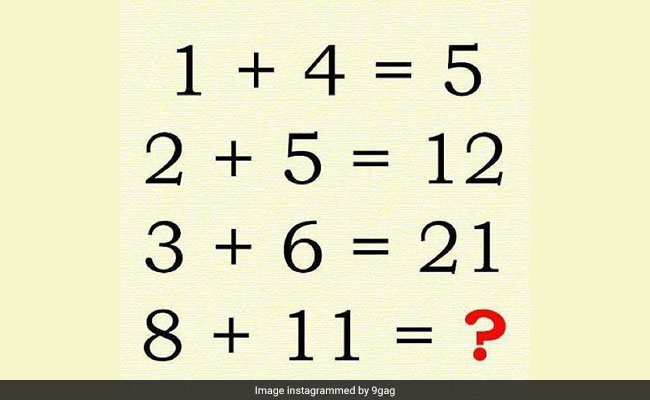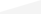Logic Puzzle With Two Answers Has The Internet Confused. Can You Solve It?

Can you figure out both the answers?

Share
EMAIL
PRINTThere are two answers to this viral puzzle. Can you figure out both?

A puzzle going viral on the Internet has everyone confused. The bizarre test, which asks you to find a pattern between four equations, has two answers - and has led to numerous heated debates online. In fact, if the Daily Mail is to be believed, only one in a 1,000 people can figure out the second answer. Go ahead and see if you can solve it:

There are two answers... Follow @9gag for mental exercises. #9gag #maths #problem #easy

A post shared by 9GAG: Go Fun The World (@9gag) on

The two answers to the puzzle are 40 and 96.

For the first solution, you begin with 1 + 4 to get 5

Then, you add 2 + 5 to the first answer of 5 to reach 12

Similarly, adding 3 + 6 to 12 gives 21

In the end, 8 + 11 + 21 = 40

The quick answer, therefore, is 40, arrived at by simply adding each equation with the sum from the previous equation.

The second answer is a little more complicated

One way to arrive at the answer of 5 in the first equation is to add 1 to (4 x 1)

Following that rule, other equations will be solved like this:

2 + (2 x 5) = 12

3 + (3 x 6) = 21

8 + (8 x 11) = 96

As is only to be expected, the puzzle has received some hate on the Internet too. However, many have pointed out that both the answers are correct since it is not a math puzzle but a logic puzzle.

Were you able to solve the question in the first try? Let us know using the comments section below.

Click for more trending news

Get Breaking news, live coverage, and Latest News from India and around the world on NDTV.com. Catch all the Live TV action on NDTV 24x7 and NDTV India. Like us on Facebook or follow us on Twitter and Instagram for latest news and live news updates.